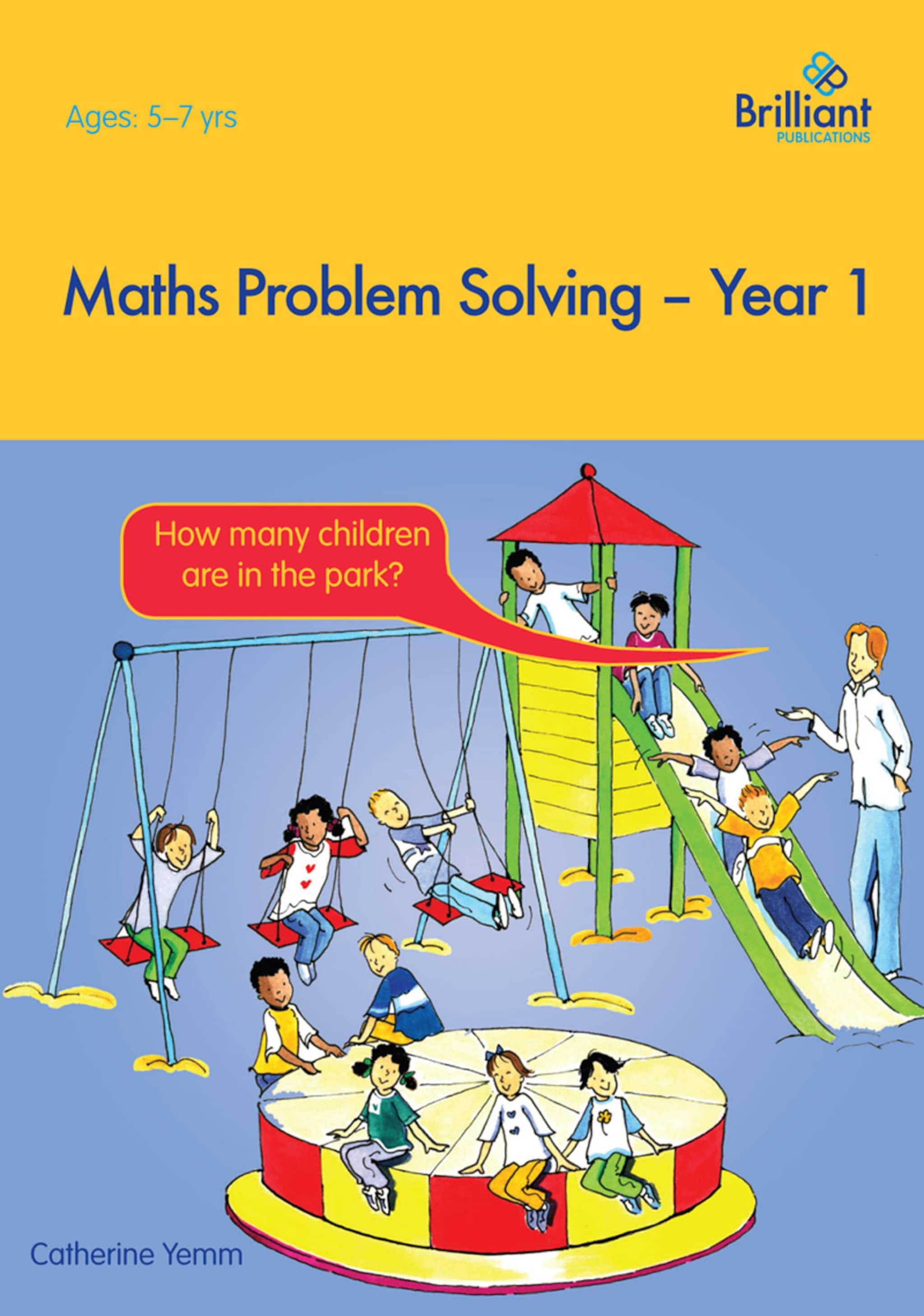Share this book
Maths Problem Solving – Year 1 is the first of six books in the Maths Problem Solving series. The books have been written for teachers to use during the numeracy lesson. They cover the ʻsolving problemʼ objectives from the numeracy framework. This first book contains four chapters; Making decisions, Reasoning about numbers or shapes, Problems involving ʻreal lifeʼ, money or measures and Organizing and using data. The books are designed in such a way that each section has six stages of questions to be worked through. Every stage is split into three levels, for example 1a, 1b or 1c, based on achievement. Each corresponding question from these levels follow the same line of questioning, so that when the teacher talks about a certain question, the solution process is the same for each level but the complexity of the sum varies.
Show more